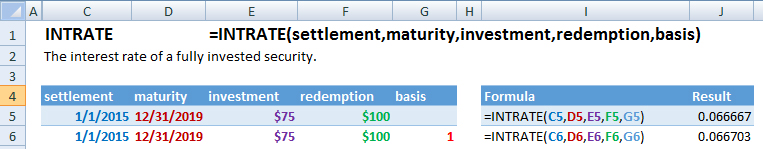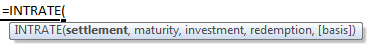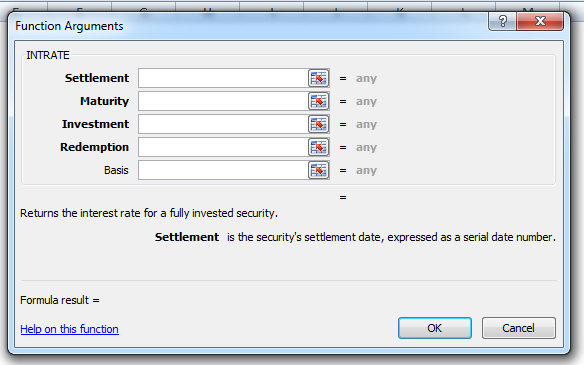### INTRATE Function Examples – Excel, VBA, & Google Sheets

This Excel Tutorial demonstrates how to use the Excel INTRATE Function in Excel to calculate the interest rate of a bond, with formula examples.

## INTRATE Function Description:

The INTRATE Function Calculates the interest rate of a fully invested security.

## Formula Examples:Example Formula Result
1 =INTRATE(C5,D5,E5,F5,G5) 6.66666666666667E-02
2 =INTRATE(C6,D6,E6,F6,G6) 0.066703196347032
AutoMacro - VBA Code Generator

## Syntax and Arguments:

The Syntax for the INTRATE Formula is:

`=INTRATE(settlement,maturity,investment,redemption,basis)`

### Function Arguments ( Inputs ):

settlement – The settlement date, represented by a date serial number.

maturity – The maturity date, represented by a date serial number.

investment – The amount invested in the security.

redemption – The redemption price per \$100 of face value.

basis – OPTIONAL. The basis for which to calculate the number of days in a year.

## Additional Notes

Use the INTRATE Function to calculate the interest rate of a bond or similar security.

The result of the INTRATE Function may appear as a decimal. Change the cell Number Formatting to Percentage to display the function result as a percentage:## INTRATE Examples in VBAYou can also use the INTRATE function in VBA. Type:
`Application.Worksheetfunction.Intrate(value,value_if_error)`
For the function arguments (value, etc.), you can either enter them directly into the function, or define variables to use instead.

Return to the List of all Functions in Excel

## How to use the INTRATE Function in Excel:

To use the AND Excel Worksheet Function, type the following into a cell:
`=AND(`
After entering it in the cell, notice how the AND formula inputs appear below the cell:You will need to enter these inputs into the function. The function inputs are covered in more detail in the next section. However, if you ever need more help with the function, after typing “=INTRATE(” into a cell, without leaving the cell, use the shortcut CTRL + A (A for Arguments) to open the “Insert Function Dialog Box” for detailed instructions:For more information about the INTRATE Formula visit the
Microsoft Website.

## Excel Practice Worksheet

Practice Excel functions and formulas with our 100% free practice worksheets!

• Automatically Graded Exercises
• Learn Excel, Inside Excel!